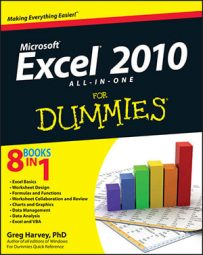##### Excel 2010 All-in-One For DummiesLinking formulas are formulas in Excel 2010 that reference a location in the same worksheet, same workbook, or even a different workbook. When you create a linking formula, it brings forward the constant or original formula to a new location so that the result in the linking formula remains dynamically tied to the original. If you change the original constant or any of the cells referred to in the original formula, the result in the cell containing the linking formula is updated at the same time as the cell containing the original constant or formula.

You can create a linking formula in one of two ways:

• Select the cell where you want to enter the linking formula, type = (equal sign), and then click the cell with the constant (text or number) or the formula that you want to bring forward to that cell. Then click the Enter button on the Formula bar or press the Enter key.

• Select the cell with the constant or formula that you want to bring forward to a new location, and click the Copy button in the Clipboard group on the Home tab or press Ctrl+C. Then click the cell where the linking formula is to appear before you click the Paste Link option on the Paste button's drop-down menu on the Home tab.

When you use the first simple formula method to create a link, Excel uses a relative cell reference to refer to the cell containing the original value or formula (as in =A10 when referring to an entry in cell A10). However, when you use the second copy-and-paste link method, Excel uses an absolute cell reference to refer to the original cell (as in =\$A\$10).

When you create a linking formula to a cell on a different sheet of the same workbook, Excel inserts the worksheet name (followed by an exclamation point) in front of the cell address. So, if you copy and paste a link to a formula in cell A10 on a different worksheet, called Income 2010, Excel inserts the following linking formula:

`='Income 2010'!\$A\$10`

When you create a linking formula to a cell in a different workbook, Excel inserts the workbook filename enclosed in brackets before the name of the worksheet, which precedes the cell address. So, if you bring forward a formula in cell A10 on a worksheet called Cost Analysis in the Projected Income 2011 workbook, Excel inserts this linking formula:

`='[Projected Income 2011.xlsx]Cost Analysis'!\$A\$10`

If you ever need to sever a link between the cell containing the original value or formula and the cell to which it's been brought forward, you can do so by editing the linking formula. Press F2, immediately recalculate the formula by pressing F9, and then click the Enter button on the Formula bar or press Enter. This replaces the linking formula with the currently calculated result. Because you've converted the dynamic formula into a constant, changes to the original cell no longer affect the one to which it was originally brought forward.